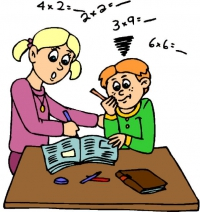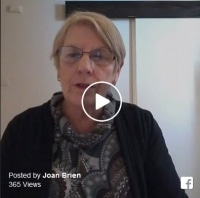Change Background:

### Blog

Helping your child understand the relationship between mathematical operations can lead to success when doing maths. For example, Multiplication and Division are related as shown in the following examples.

Multiplication gives an answer that is “greater than” the number you start with in the question. E.g., “12 x 6 = 72” and “6 x 12 = 72”. Multiplying makes a “bigger number” than either “12” or “6”.

## The Relationship Between Multiplication and Division

Division gives an answer that is smaller than the number that you started off with in the question. E.g., “72 ÷ 12 = 6” and “72 ÷ 6 = 12”. Division makes a “smaller number” than 72.

The above three numbers (6,12 and 72) are related (as shown above), so if your child can see this relationship, it can help them to work out problems that involve these three numbers.

Another way to think of the relationship between multiplication and division is that they are the “reverse of each other”.

This and other helpful hints for parents can be found in my book ‘Can I Help My Child Learn? A Parent Guide Written in Plain English”Rate this This Item
5/5Uncategorized### THE TREATMENT FOR IRLEN SYNDROME

The treatment for Irlen Syndrome is NOT a programme but IS a method to help visual processing dysfunction. Many critics of Irlen suggest that if